UPDATE: At the bottom are two additional methods, and some additions (underlined) are added to the original 5 methods. Thanks for all the feedback...

-Also, another post here about ordered-categorical data
-Also #2, a method combining splom and hexbin packages here, for larger datasets

In data analysis it is often nice to look at all pairwise combinations of continuous variables in scatterplots. Up until recently, I have used the function splom in the package lattice, but ggplot2 has superior aesthetics, I think anyway.

Here a few ways to accomplish the task:

`require(lattice)require(ggplot2) `
`require(car)`

1) Using base graphics, function “pairs”

`pairs(iris[1:4], pch = 21)`

Created by Pretty R at inside-R.org

<div class="separator" style="clear: both; text-align: center;">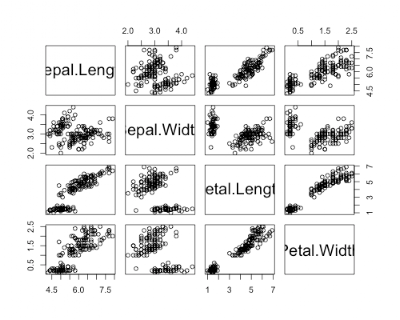</div>

2) Using lattice package, function “splom”

-Additional code to improve splom plots here (and see Oscar's code below in comments)

<div style="overflow: auto;"><div class="geshifilter"><pre class="r geshifilter-R" style="font-family: monospace;">splom(~iris[1:4])</pre></div></div>Created by Pretty R at inside-R.org

<div class="separator" style="clear: both; text-align: center;">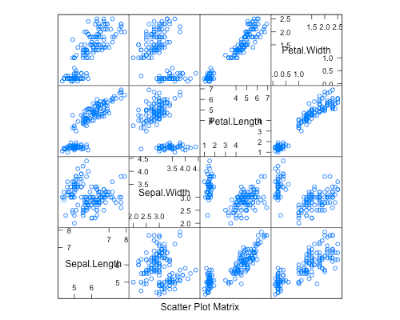</div>

3) Using package ggplot2, function “plotmatrix”

<div style="overflow: auto;"><div class="geshifilter"><pre class="r geshifilter-R" style="font-family: monospace;">plotmatrix(iris[1:4])</pre></div></div>Created by Pretty R at inside-R.org

<div class="separator" style="clear: both; text-align: center;">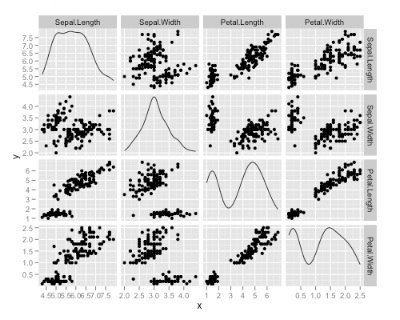</div>

4) a function called ggcorplot by Mike Lawrence at Dalhousie University

-get ggcorplot function at this link
-ggcorplot is also built in to Deducer (get here); see Ian's code below in the comments
-Lastly, an improved version of ggcorplot is built in to the ez package (get here)

<div style="overflow: auto;"><div class="geshifilter"><pre class="r geshifilter-R" style="font-family: monospace;">ggcorplot(
data = iris[1:4],
var_text_size = 5,
cor_text_limits = c(5,10))</pre></div></div>Created by Pretty R at inside-R.org

<div class="separator" style="clear: both; text-align: center;">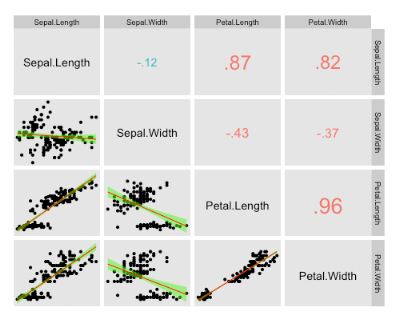</div>

5) panel.cor function using pairs, similar to ggcorplot, but using base graphics. Not sure who wrote this function, but here is where I found it.
<div style="overflow: auto;"><div class="geshifilter"><pre class="r geshifilter-R" style="font-family: monospace;"></pre></div></div><div style="overflow: auto;"><div class="geshifilter"><pre class="r geshifilter-R" style="font-family: monospace;">panel.cor <- function(x, y, digits=2, prefix=””, cex.cor)
{
usr <- par(“usr”); on.exit(par(usr))
par(usr = c(0, 1, 0, 1))
r <- abs(cor(x, y))
txt <- format(c(r, 0.123456789), digits=digits)
txt <- paste(prefix, txt, sep=””)
if(missing(cex.cor)) cex <- 0.8/strwidth(txt)

test <- cor.test(x,y)
# borrowed from printCoefmat
Signif <- symnum(test\$p.value, corr = FALSE, na = FALSE,
cutpoints = c(0, 0.001, 0.01, 0.05, 0.1, 1),
symbols = c(“***”, “**”, “*”, ”.”, ” “))

text(0.5, 0.5, txt, cex = cex * r)
text(.8, .8, Signif, cex=cex, col=2)
}
</pre><pre class="r geshifilter-R" style="font-family: monospace;">pairs(iris[1:4], lower.panel=panel.smooth, upper.panel=panel.cor)</pre></div></div>Created by Pretty R at inside-R.org
<div class="separator" style="clear: both; text-align: center;">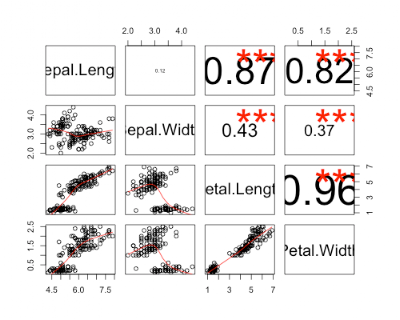</div>

A comparison of run times…

<div style="overflow: auto;"><div class="geshifilter"><pre class="r geshifilter-R" style="font-family: monospace;">> system.time(pairs(iris[1:4]))
user system elapsed
0.138 0.008 0.156
> system.time(splom(~iris[1:4]))
user system elapsed
0.003 0.000 0.003
> system.time(plotmatrix(iris[1:4]))
user system elapsed
0.052 0.000 0.052
> system.time(ggcorplot(
+ data = iris[1:4],
var_text_size = 5,
cor_text_limits = c(5,10)))

user system elapsed
0.130 0.001 0.131
> system.time(pairs(iris[1:4], lower.panel=panel.smooth, upper.panel=panel.cor))
user system elapsed
0.170 0.011 0.200</pre></div></div>Created by Pretty R at inside-R.org

…shows that splom is the fastest method, with the method using the panel.cor function pulling up the rear.

6) given by a reader in the comments (get her/his code here). This one is nice as it gives 95% CI’s for the correlation coefficients, AND histograms of each variable.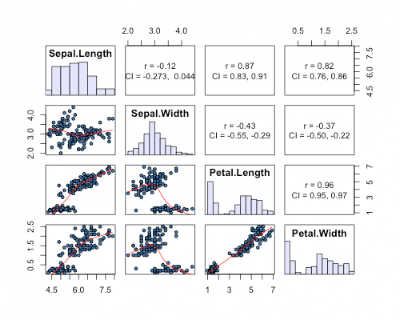7) a reader in the comments suggested the scatterplotMatrix (spm can be used) function in the car package. This one has the advantage of plotting distributions of each variable, and providing fits to each data with confidence intervals.

<div style="overflow: auto;"><div class="geshifilter"><pre class="r geshifilter-R" style="font-family: monospace;">spm(iris[1:4])</pre></div></div>

<div class="separator" style="clear: both; text-align: center;">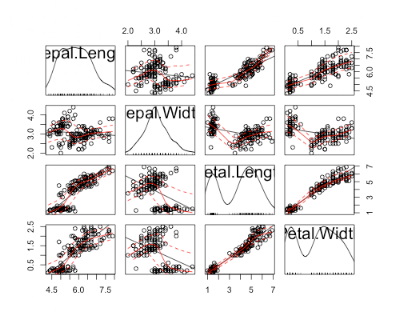</div>
<div class="separator" style="clear: both; text-align: center;">

</div>